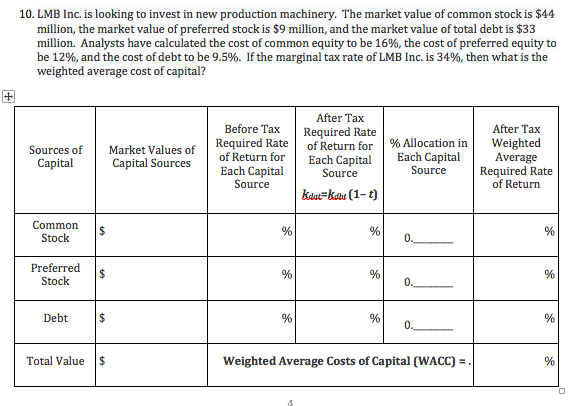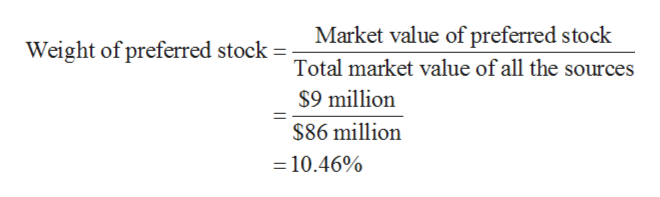# 10. LMB Inc. is looking to invest in new production machinery. The market value of common stock is \$44million, the market value of preferred stock is \$9 million, and the market value of total debt is \$33million. Analysts have calculated the cost of common equity to be 16%, the cost of preferred equity tobe 12%, and the cost of debt to be 9.5%. If the marginal tax rate of LMB Inc. is 34%, then what is theweighted average cost of capital?After TaxBefore TaxAfter TaxRequired Rateof Return forRequired Rateof Return for% Allocation inEach CapitalSourceWeightedAverageRequired Rateof ReturnSources ofMarket Values ofEach CapitalSourceCapitalCapital SourcesEach CapitalSourcekdat-kabr (1-t)CommonStock0.Preferred\$Stock0.Debt\$0.Weighted Average Costs of Capital (WACC)Total Value\$206

Question
13 viewshelp_outlineImage Transcriptionclose10. LMB Inc. is looking to invest in new production machinery. The market value of common stock is \$44 million, the market value of preferred stock is \$9 million, and the market value of total debt is \$33 million. Analysts have calculated the cost of common equity to be 16%, the cost of preferred equity to be 12%, and the cost of debt to be 9.5%. If the marginal tax rate of LMB Inc. is 34%, then what is the weighted average cost of capital? After Tax Before Tax After Tax Required Rate of Return for Required Rate of Return for % Allocation in Each Capital Source Weighted Average Required Rate of Return Sources of Market Values of Each Capital Source Capital Capital Sources Each Capital Source kdat-kabr (1-t) Common Stock 0. Preferred \$ Stock 0. Debt \$ 0. Weighted Average Costs of Capital (WACC) Total Value \$ 206 fullscreen
check_circle

Step 1

Hence, weight of equity is 51.16%, which is determined by dividing the equity market value with the total of all sources market value.

Step 2

Hence, weight of preferred stock is 10.46%, which is determined by dividing the preferred stock market value with the total of all sources market value.help_outlineImage TranscriptioncloseMarket value of preferred stock Weight of preferred stock Total market value of all the sources \$9 million \$86 million =10.46% fullscreen
Step 3

Hence, weight of debt is 51.16%, which is determined by dividing the ...

### Want to see the full answer?

See Solution

#### Want to see this answer and more?

Solutions are written by subject experts who are available 24/7. Questions are typically answered within 1 hour.*

See Solution
*Response times may vary by subject and question.
Tagged in